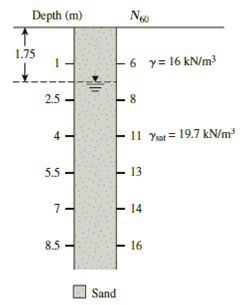Chapter 17, Problem 17.8PPrinciples of Geotechnical Enginee...

9th Edition
Braja M. Das + 1 other
ISBN: 9781305970939

Solutions

Chapter
SectionPrinciples of Geotechnical Enginee...

9th Edition
Braja M. Das + 1 other
ISBN: 9781305970939
Textbook Problem

Refer to Problem 17.7 and Figure 17.16. Suppose a footing (1.5 m × 1.5 m) is constructed at a depth of 1 m.a. Estimate the design values for N60 and ϕ ′ .b. What is the net allowable load that the footing can carry? The maximum allowable 17.7 settlement is 25 mm. Use Eqs. (16.56) and (16.61).Refer to the boring log shown in Figure 17.16. Estimate the average drained friction angle, ϕ ′ , using the Kulhawy and Mayne correlation [Eq. (17.24)]. Assume pa ≈ 100 kN/m2.Figure 17.16

(a)

To determine

Find the design value for N60 and ϕ.

Explanation

Given information:

The allowable settlement is 25mm.

The atmospheric pressure is (pa) 100kN/m2.

Assume the average unit weight (γ) of sand is 16kN/m3.

The footing size is (1.5×1.5)m.

The depth of footing (Df) is 1m.

The saturated (γsat) unit weight is 19.7kN/m3.

Show the depth and number of blows shown in table:

 Depth,(m) N60 1 6 2.5 8 4 11 5.5 13 7 14 8.5 16

Calculation:

Calculate the design value for N60 using the relation.

The properties should be averaged over a distance 2B or 3m below the footing up to 4m

Calculate the design value for N60 using the Figure.

N60=(6+8+11)3=253=8.338

Find the effective overburden pressure (σo) at depth of 1m using the relation:

σo=Hγ (1)

Here, H1 is depth of 1m.

Substitute 1m for H1 and 16kN/m3 for (γ) in Equation (1).

σo=1×16=16kN/m2

Find the effective overburden pressure (σo) at depth of 2.5m using the relation:

σo=H1γ+H2(γsatγw) (2)

Here, γw is unit weight of water.

Substitute 1.75m for H1, 19.7kN/m3 for (γsat),9.81kN/m3 for γw, (1.751)m for H2, and 16kN/m3 for (γ) in Equation (2).

σo=1.75×16+(1.751)(19.79.81)=28+7.4175=35.41735.42kN/m2

Find the effective overburden pressure (σo) at depth of 4m using the relation:

σo=H1γ+(H2)(γsatγw) (3)

Substitute 1

(b)

To determine

Find the net allowable load such that the foot can carry.

Still sussing out bartleby?

Check out a sample textbook solution.

See a sample solution

The Solution to Your Study Problems

Bartleby provides explanations to thousands of textbook problems written by our experts, many with advanced degrees!

Get Started

Find the rectangular representation of the force F, given that its magnitude is 320 N.

International Edition---engineering Mechanics: Statics, 4th Edition

What is the purpose of a benchmark test? Suggest at least two examples of benchmarks.

Systems Analysis and Design (Shelly Cashman Series) (MindTap Course List)

How can cutting torch tip seals be repaired?

Welding: Principles and Applications (MindTap Course List)